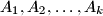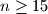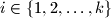IMO Shortlist 2011 problem C4

Kvaliteta:
Avg: 0,0
Težina:
Avg: 7,0
Determine the greatest positive integer$k$ that satisfies the following property: The set of positive integers can be partitioned into$k$ subsets$A_1, A_2, \ldots, A_k$ such that for all integers$n \geq 15$ and all$i \in \{1, 2, \ldots, k\}$ there exist two distinct elements of$A_i$ whose sum is$n.$

Proposed by Igor Voronovich, Belarus
Izvor: Međunarodna matematička olimpijada, shortlist 2011